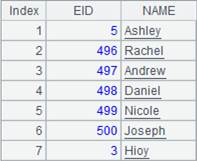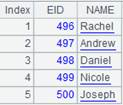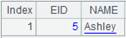# channel()

## channel()

 channel ()

 A 1 =demo.cursor("select * from SCORES where CLASS = 'Class one'") 2 =channel() 创建管道 3 =A1.push(A2) 将游标A1中的数据推送到管道A2， 此时数据不会立即被推送到管道 4 =channel(A1) 相当于表达式:=A1.push(channel())，将游标A1中的数据推送到管道，此时数据不会立即被推送到管道，结果返回管道 5 =channel(A2) 将管道A2中的数据推送到该管道中，结果返回管道

## channel(cs)

channel(cs)

 cs 游标

 A 1 =demo.cursor("select * from SCORES where CLASS = 'Class one'") 2 =channel(A1) 创建管道后将游标A1中的数据推送到管道，此时数据不会立马被推送到管道，相当于表达式:=A1.push(channel())。结果返回管道。 3 >A2.select(STUDENTID==1) 4 >A2.fetch() 5 =A1.fetch() 6 =A2.result()## channel(cs)

channel(cs)

 cs 游标

 A 1 =file("emp1.ctx":,"192.168.0.119:8281") 2 =A1.open() 打开集群表 3 =A2.attach(table1) 取出集群附表 4 =A3.memory() 创建集群内表 5 =A4.cursor() 返回集群游标 6 =channel(A5) 将游标中的数据推送到管道，此时数据不会立即被推送到管道，结果返回集群管道 7 =A6.fetch() 保留集群管道中的数据 8 =A5.fetch() 9 =A6.result() 获取集群管道计算结果

## channel(ch)

channel(ch)

 ch 管道

 A 1 =demo.cursor("select * from SCORES where CLASS = 'Class one'") 2 =channel() 创建管道 3 =channel(A2) 创建管道并且将管道A2中的数据推送到管道A3，相当于表达式:=A2.push(channel()) 4 >A2.select(STUDENTID==1) 5 >A3.select(STUDENTID==2) 6 =A2.fetch() 7 =A3.fetch() 8 =A1.push(A2) 9 =A1.fetch() 10 =A2.result()11 =A3.result()## channel(ch)

channel(ch)

 ch 集群管道

 A 1 =file("emp1.ctx":,"192.168.0.119:8281") 2 =A1.open() 打开集群表 3 =A2.attach(table1) 取出集群实表table1 4 =A3.cursor() 返回集群游标 5 =channel(A4) 将集群游标中的数据推送到集群管道，此时数据不会立马被推送到集群管道，结果返回集群管道 6 =channel(A5) 将集群管道中的数据推送到集群管道，此时数据不会立马被推送到集群管道，结果返回集群管道 7 =A5.fetch() 保留集群管道中的数据 8 =A6.fetch() 保留集群管道中的数据 9 =A4.fetch() 10 =A5.result() 获取集群管道计算结果 11 =A6.result() 获取集群管道计算结果